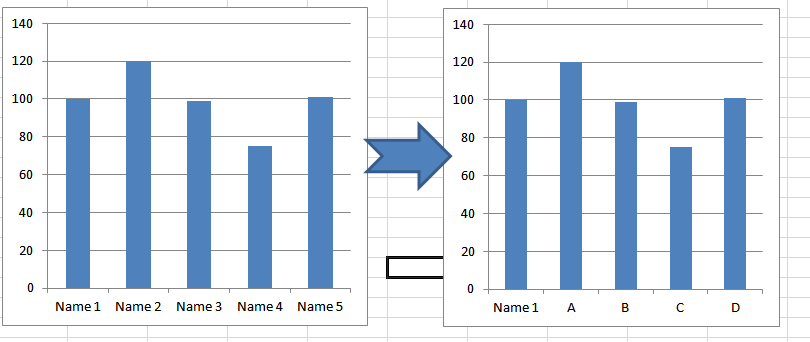QlikView Layout & Visualizations

Discussion Board for collaboration on QlikView Layout & Visualizations.

HighlightedNot applicable

How to create dynamic Dimension Label in Charts?

Dear Colleagues,

I'm trying to create into a Bar Charts dynamic name creation in the Dimension field.

Basically, I want to keep the original value for the "Name 1" first position and them for the remaining this should be visible other names as A, B, C, D depending on number of dimensions.

Please see below in the picture below the desired results:I appreciate any good idea on how to create this calculated dimension

Thanks! Leandro

Tags (3)
9 Replies
Valued Contributor

Re: How to create dynamic Dimension Label in Charts?

When you say "depending on the number of dimensions" - what are ABCD representing?Not applicable

Re: How to create dynamic Dimension Label in Charts?

Hi, this is dynamic. it can be many depending on selection.

I would say it is never more than 5

Valued Contributor

Re: How to create dynamic Dimension Label in Charts?

What I mean is, are these values of a dimension, or are these dimensions themselves? It might help if you give an example of a situation alluding to what sort of data you're talking aboutNot applicable

Re: How to create dynamic Dimension Label in Charts?

Hi,

My database contains the Name 1, Name 2, Name 3 and I cannot simple define the Name 1 = A, Name 2 = B since position in the chart change and I want to keep a sequence A,B,C...

In my view this should be calculated dimension depending on the Position in the chart: i.e. if this is position is 1 = Name 1, if position is 2 = "A", if position is 3 = "B"... something like this.

Since the position of each "Name" is very dynamic, I believe I should have some calculated dimension.Partner

Re: How to create dynamic Dimension Label in Charts?

Hi,

Can you please provide a small dataset of your application to have a clear understanding.

Thanks and Regards,

Ankita

Valued Contributor

Re: How to create dynamic Dimension Label in Charts?

There may be other ways around it, but one way would be:

> Create a calculated dimension that uses ValueList (for example: ValueList('Name 1', 'A', 'B')

> Create a conditional expression to return your dimension value based on your expression value, for example:

=If(ValueList('Name 1', 'A', 'B')='Name 1', Sum({<Year={"=Rank(Sum(Sales))=1"}>}Sales),
If(ValueList('Name 1', 'A', 'B')='A', Sum({<Year={"=Rank(Sum(Sales))=2"}>}Sales),
Sum({<Year={"=Rank(Sum(Sales))=3"}>}Sales)))

This is an example that instead of returning the year, would return the values 'Name 1', 'A' and 'B' in order of rank and attribute an individual years sales to each bar in size order. It's worth noting these wouldn't be 'clickable' though, as it's a ValueList and not the true values, so I'm not sure if that's exactly what you're after?Not applicable

Re: How to create dynamic Dimension Label in Charts?

Hi, I have added the qlikview file in the first message

Valued Contributor

Re: How to create dynamic Dimension Label in Charts?

See attached for an example of what I said below.Not applicable

Re: How to create dynamic Dimension Label in Charts?

Please try like the below expression

=If(Aggr(Rank(Sum(Sales)),Customer)<=10,'A','B')